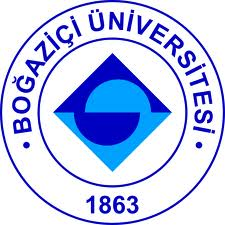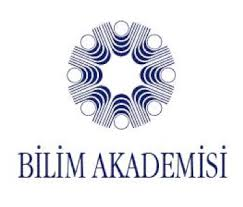Home Speakers Program Practical Info Organizers

# Recent Developments in Supergravity Theories and Related Topics

## This workshop is dedicated to the memory of Prof. Rahmi Güven (1948 – 2019).

Speakers (all titles and abstracts)

• Gleb Arutyunov, Hamburg University
• Generalized Supergravity

I will explain how the studies of integrable deformations of the AdS_5 x S^5 string sigma model led to the discovery of the generalized supergravity.
• Aybike Çatal-Özer, İstanbul Technical University
• Double Field Theory and Integrable Deformations of String Sigma Models

We show that Non–Abelian T–duality (NATD) and Yang–Baxter (YB) deformation of string sigma models can both be described as a coordinate dependent O(d,d) transformation. Our approach gives a natural embedding of the NATD and the homogeneous YB model in Double Field Theory (DFT), a framework which provides an O(d,d) covariant formulation for effective string actions. We show that the deformed fields can be regarded as duality twisted fields in the context of Gauged Double Field Theory. We compute the fluxes associated with the twist and show that the conditions on the R–matrix determining the YB deformation give rise to conditions on the fluxes on the (G)DFT side. We also discuss how the non–unimodularity of the symmetry algebra forces the generalized dilaton field to pick up a linear dependence on the winding type coordinates of DFT, implying that the corresponding supergravity fields should satisfy generalized supergravity equations.
• Ömer Faruk Dayı, İstanbul Technical University
• Chiral Kinetic Theory in the Rotating Frame

A modified quantum kinetic equation which takes account of the noninertial features of rotating frame is introduced. In terms of the axial components of Wigner functions covariant chiral kinetic theory is obtained. It uniquely provides a new three-dimensional semiclassical chiral kinetic theory which is independent of spatial coordinates. The particle number and current densities deduced from this transport equation satisfy the anomalous continuity equation and generate the magnetic and vortical effects correctly.
• Cemsinan Deliduman, Mimar Sinan University
• Gravitational Lensing in Weyl Gravity

We will review our calculation of the deflection angle of light from a distant source by a galaxy cluster in Weyl’s conformal gravity. The general method of calculation will first be applied to calculate the deflection angle in Schwarzschild–de Sitter (Kottler) spacetime. The deflection angle calculated in Kottler spacetime will be seen to include the contribution of the cosmological constant. We will then calculate the deflection angle in Mannheim-Kazanas spacetime and show that the result includes contributions from both the cosmological constant and the Mannheim-Kazanas parameter. There are conflicting results on the deflection angle for light in Weyl gravity in the literature. We will point out a possible reason for the discrepancy between our work and the others.
• Durmuş Ali Demir, Sabancı University
• Gauge Invariance and QFT-GR Blend

In QFTs in flat spacetime, gauge invariance is explicitly broken by the Poincare breaking scale. It will be shown in this talk that GR can be incorporated into the QFT in a way restoring gauge invariance and thereby completing the QFT in the UV.
• Hovhannes Demirchian, Yerevan Physics Institute
• Integrability of Geodesics in Near-horizon Extremal Myers-Perry Black Holes

We study the first integrals of probe particles moving in the near-horizon limit of extremal Myers-Perry black holes in arbitrary dimensions. We show that this problem is integrable and separable. We find the general solution of the Hamilton-Jacobi equations for these systems and present explicit expressions for the Liouville integrals as well as discuss Killing tensors and the associated constants of motion. We analyze special cases of the background near-horizon geometry where the system is superintegrable as well as consider near-horizon extremal vanishing horizon geometry.
• Tekin Dereli, Koç University
• A class of Exact Solutions of Supersymmetric Yang-Mills Equations

We give a class of solutions to supersymmetric Yang-Mills field equations (i) that cannot be generated from solutions of the ordinary Yang-Mills equations by finite supersymmetry transformations and (ii) contains the supersymmetric generalization of non-Abelian plane waves. (This talk is based on the following joint paper with Rahmi Güven: Phys.Lett. 84B (1979) 201-204.)
• Tigran Hakobyan, Yerevan State University
• Symmetry Algebra of Dynamical and Discrete Calogero Models

The symmetries of the N-dimensional (generalized) dynamical Calogero model and its discrete version are related to the well-known symmetries of the N-dimensional isotropic oscillator given by the U(N) group. The correspondence is provided by the Dunkl-operator deformation of the usual quantum momentum.
• Exactness of the Born Approximation and Broadband Unidirectional Invisibility

Born approximation was proposed by Max Born in his monumental 1926 paper where he laid the foundations of quantum scattering theory. Because of its great theoretical and practical significance, this approximation scheme is covered in most standard textbooks on quantum mechanics. None of these, however, addresses the problem of finding potentials whose scattering problem is exactly solvable by the Born approximation. We give a simple condition under which a potential has this property in two dimensions. This condition identifies a very large class of potentials. This in turn allows us to solve another important open problem of scattering theory, namely constructing potentials that enjoy perfect (non-approximate) unidirectional invisibility in a finite spectral band of arbitrary width. Our analysis provides the first examples of quasi-exactly solvable scattering potentials, i.e., potentials whose scattering problem is exactly solvable for energies not exceeding a given value.
• Özgür Sarıoğlu, Middle East Technical University
• Circularly Symmetric Solutions of Minimal Massive Gravity at Its Merger Point

I find all the static circularly symmetric solutions of minimal massive 3D gravity at its merger point, construct stationary versions of these and discuss some of their geometric and physical properties. It turns out that apart from a static hairy black hole, there is also a static gravitational soliton, that has been overlooked in the literature.
• Hovhannes Shmavonyan, Yerevan Physics Institute
• Symmetries of Deformed Supersymmetric Mechanics on Kahler Manifolds

We construct the deformed N=4,8 supersymmetric mechanics on Kahler manifolds interacting with constant magnetic field, and study their symmetries. At first we construct the deformed N=4,8 supersymmetric Landau problem. We show that the initial ’flat’ supersymmetries are necessarily deformed to SU(2∣1) and SU(4∣1) supersymmetries, with the magnetic field playing the role of deformation parameter, and that the resulting systems inherit all the kinematical symmetries of the initial ones. Then we construct SU(2∣1) supersymmetric Kahler oscillators and find that they include, as particular cases, the harmonic oscillator models on complex Euclidean and complex projective spaces, as well as superintegrable deformations of C^N–Smorodinsky–Winternitz and CP^N–Rosochatius systems. We show that the supersymmetric extensions proposed inherit all the kinematical symmetries of the initial bosonic models. They also inherit, at least in the case of C^N systems, hidden (non–kinematical) symmetries.
• Stepan Sidorov, Bogoliubov Laboratory of Theoretical Physics
• Deformed Models of Supersymmetric Quantum Mechanics

We study deformed models of supersymmetric quantum mechanics constructed in the framework of SU(2∣1) superfield approach, where the relevant superalgebra su(2∣1) is defined as a deformation of the standard N=4, d=1 Poincare superalgebra by a mass dimensional parameter m. Due to this parameter, SU(2∣1) supersymmetric models contain additional deforming terms corresponding to the magnetic field and oscillator potentials. We perform quantization with the construction of Hilbert space of wave functions. Some peculiarities of the quantum spectra find a natural explanation in the framework of SU(2∣1) representation theory.
• Bayram Tekin, Middle East Technical University
• Non-Stationary Energy in Gravity

Quantification of the amount of gravitational radiation in a given spacetime is an important problem. Following Dain's ideas, we shall give an integral that measures this energy in a given spatial hypersurface in spacetime. (This talk is based on a joint work with E. Altas)
• George Thompson, Ictp
• Chern-Simons Theory on a General Seifert 3-Manifold

The path integral for the partition function of Chern-Simons gauge theory with a compact gauge group is evaluated on a general Seifert 3-manifold. This extends previous results and relies on abelianisation, a background field method and local application of the Kawasaki Index theorem.